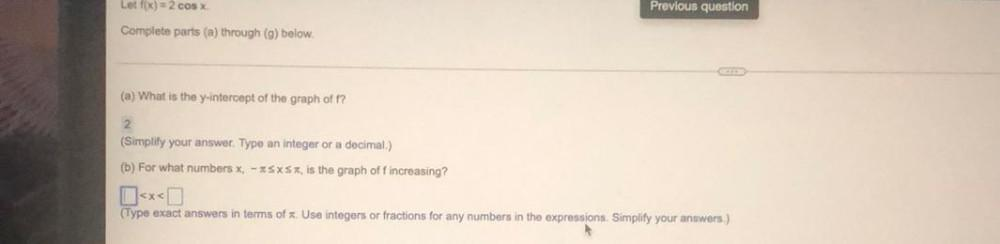Question:

# Lot f(x) = 2 cos x Previous question Complete parts (a) through (9) below. (a) What is the y-intercept of the graph off? 2. (SimLot f(x) = 2 cos x Previous question Complete parts (a) through (9) below. (a) What is the y-intercept of the graph off? 2. (Simplify your answer. Type an integer or a decimal.) (b) For what numbers x, -*SXSX, is the graph off increasing? (Type exact answers in terms of Use integers or fractions for any numbers in the expressions. Simplify your answers.)